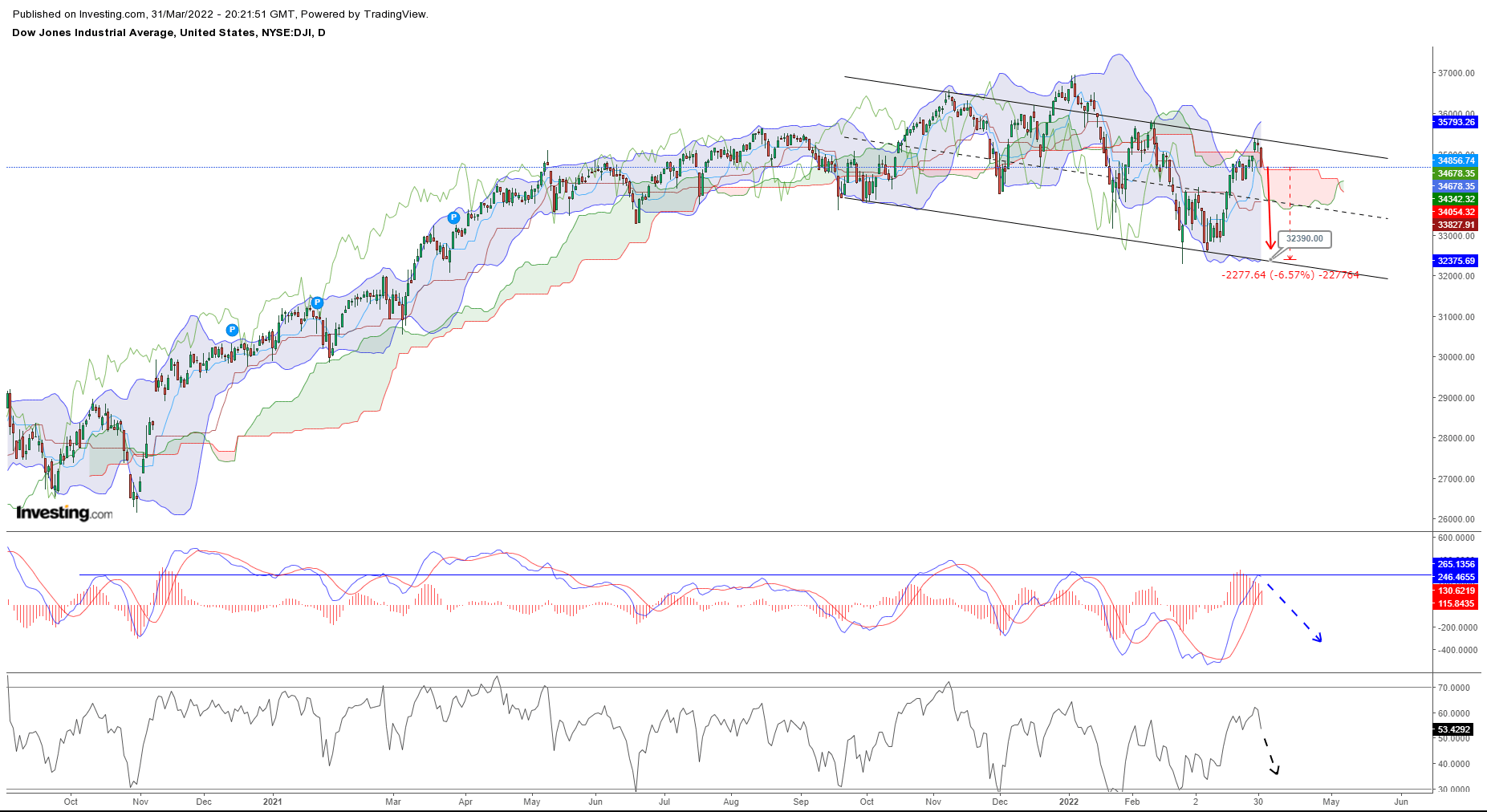# Dow Industrial’s Bollinger band technical signal of drift lower to Dow 32390

Mar 31, 2022Views: 570

The leveraged gains in the Dow30 index and listed stocks in statistical terms have been achieved with negative returns skewness, that means the standardization of returns are skewed in the left tail of the distribution, in fact, basic data as the Dow30 mean value being smaller than the median and the mode, the basics do prove that the skewness of returns, i.e. cumulative %changes, are negative or the standardization of return with the Gaussian value 0.00107, derived by the DOW30 data, does imply a -3σ(Sigma) volatility.

When considering the Dow Jones Industrial Average trendline, the function f(x)=e^-x^2, that describes the exponential distribution of indexes and other stock markets instruments that have a Gaussian distribution. Of course, if we pretend to calculate the Area underneath the Trendline, by Integration of the exponential function, in mathematical terms the exponential function can be integrated, √πerf(x)/2. Exponential functions are of a particular kind, that in fact the exponential function does produce the Gaussian error function (erf), in other words, exponential function and distribution do always respond to Standard normal distribution, in fact, Integrating the exponential function does produce the Gauss error function, or the standardization of the error, in terms of deviation from the mean derived by an exponential function, that ends up being a standard normal distribution of returns.